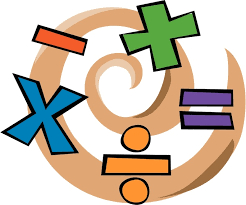# Illustrate

Illustrate the following expressions then solve.
1. 3-8/10=
2. 4-2/5=
3. 2-3/8=
4. 3-3/7=
5. 5-4/9=

a =  11/5 = 2 15
b =  18/5 = 3 35
c =  13/8 = 1 58
d =  18/7 = 2 47
e =  41/9 = 4 59

### Step-by-step explanation:Did you find an error or inaccuracy? Feel free to write us. Thank you!

Tips to related online calculators
Need help to calculate sum, simplify or multiply fractions? Try our fraction calculator.